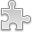# Courses / Module

Toggle Print

##FOUNDATIONS OF EUCLIDEAN GEOMETRY

Module code: MT251P
Credits: 5
Semester: 1
Department: MATHEMATICS AND STATISTICS
International:Overview

Overview:
Problem solving � analysis of mathematical behavior. Proof �Mathematical notation. Basic notions from formal logic. Nature and techniques of proof. Counterexamples.

Euclid's postulates. Fundamental theorems in Euclidean Geometry. Vectors; dot and cross products. Basics of matrix algebra and its applications to geometry; determinants, inverse matrices, linear algebra and row reduction.

Other topics may include:
Lines and planes; conic sections. Positive definite quadratic forms in two variables.Learning OutcomesTeaching & Learning methodsAssessmentAutumn Supplementals/ResitsPre-RequisitesTimetable